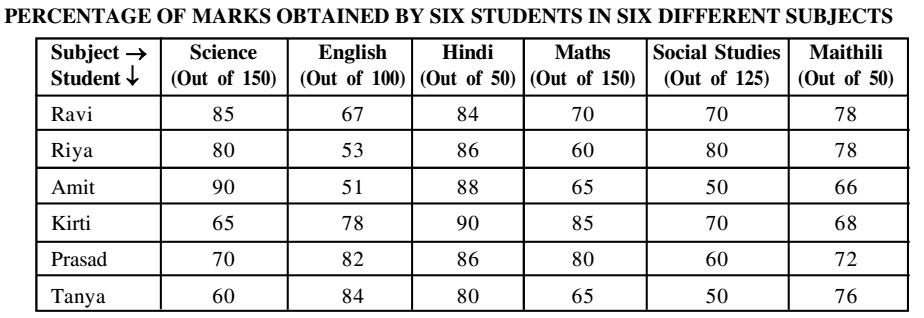Home » Data Interpretation » Table chart » Question

#### Data Interpretation

Direction: Study the table carefully to answer the questions that follow:1. What are the average marks obtained by all students together in Hindi?
1. 41.54
2. 42.83
3. 43.28
4. 44.62
##### Correct Option: B

As per given table graph,
The total marks obtained by all students in Hindi = Marks obtained by ravi in Hindi + Marks obtained by riya in Hindi + Marks obtained by amit in Hindi +Marks obtained by kirti in Hindi + Marks obtained by prasad in Hindi + Marks obtained by tanya in Hindi
The total marks obtained by all students in Hindi = 50 x (84 + 86 + 88 + 90 + 86 + 80)/100 = 50 x 514/100 = 257
The average marks obtained by all students in Hindi = 257/6 = 42.83